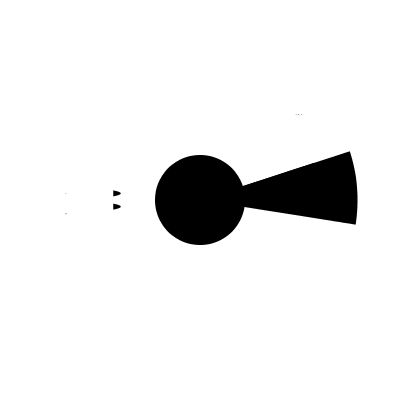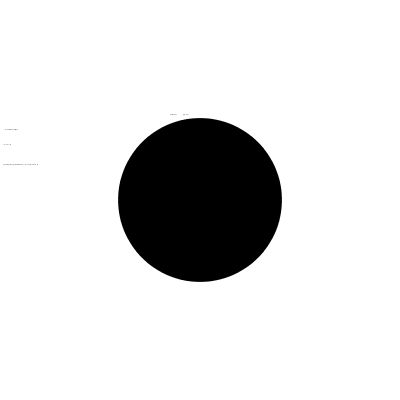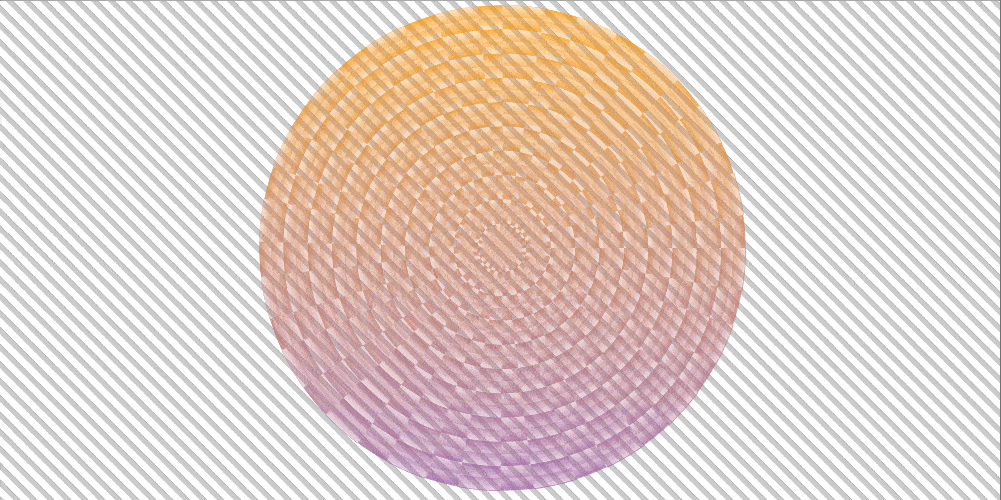2018
04-25

# 一个HTML元素和五个CSS属性的魔力## CSS中的渐变射线

`<div class='rays'></div>`

```.rays {
width: 80vmin;
height: 80vmin;
}``````\$nr: 20;          // 射线数量
\$p: 100% / \$nr;   // 射线和间距所占圆的百分比

.rays {
/* 和前面相同的几个样式 */
}```Webkit内核浏览器中的 mask 仍然需要添加 -webkit- 前缀。你会认为这没问题，因为我们使用的是Polyfill，而它依赖于 -prefix-free ，所以，如果我们使用Polyfill，我们需要在它之前引入 -prefix-free 。不幸的是，这比我们想的要复杂一点。主要是因为 -prefix-free 的运行需要通过特性检测，而在这种情况下常会失败，这是因为所有的浏览器都支持SVG不带前缀的 mask 属性。但是我们在HTML元素上使用了 mask ，而Wekit内核浏览器又需要 -webkit- 前缀的情部下， -prefix-free 又不会添加，所以需要手动去添加：

```\$nr: 20;
\$p: 100%/\$nr;
\$m: repeating-conic-gradient(#000 0% .5*\$p, transparent 0% \$p);

.rays {
}```

### 添加动画

```\$m: repeating-conic-gradient(
rgba(#000, var(--a)) 0% .5*\$p,
rgba(#000, calc(1 - var(--a))) 0% \$p);```

```CSS.registerProperty({
name: '--a',
syntax: '<number>',
initialValue: 1;
})```

```.rays {
animation: a 2s linear infinite alternate;
}

@keyframes a {
to {
--a: 0
}
}``````\$m: repeating-conic-gradient(
rgba(#000, var(--a0)) 0%, rgba(#000, var(--a1)) .5*\$p,
rgba(#000, var(--a2)) 0%, rgba(#000, var(--a3)) \$p);```

```for(let i = 0; i < 4; i++) {
CSS.registerProperty({
name: `--a\${i}`,
syntax: '<number>',
initialValue: 1 - ~~(i/2)
})
}```

```.rays {
animation: a 2s infinite alternate;
animation-name: a0, a1, a2, a3;
animation-timing-function:
cubic-bezier(.57, .05, .67, .19) /* easeInCubic */,
cubic-bezier(.21, .61, .35, 1); /* easeOutCubic */
}

@for \$i from 0 to 4 {
@keyframes a#{\$i} {
to {
--a#{\$i}: #{floor(\$i/2)}
}
}
}````\$m: repeating-conic-gradient(#000 0% var(--p), transparent 0% \$p);`

```CSS.registerProperty({
name: '--p',
syntax: '<percentage>',
initialValue: '0%'
})```

```.rays {
animation: p .5s linear infinite alternate
}

@keyframes p {
to {
--p: #{\$p}
}
}``````\$t: .5s;

.rays {
animation: p \$t linear infinite alternate, s 2*\$t steps(1) infinite;
}

@keyframes p {
to {
--p: #{\$p}
}
}

@keyframes s {
50% {
transform: scalex(-1);
}
}```## 渐变射线和波纹```\$nr: 20;
\$p: 100% / \$nr;
\$stop-list: #000 0% .5*\$p, transparent 0% \$p;

.rays-ripples {
}```

```\$nr: 20;
\$p: 100% / \$nr;
\$stop-list: #000, #000 .5*\$p, transparent 0%, transparent \$p;

.rays-ripples {
}``````\$m: repeating-conic-gradient(\$stop-list) exclude,### 添加动画

```// for conic gradient
\$nc: 20;
\$pc: 100%/\$nc;

\$nr: 10;
\$pr: 100%/\$nr;```

```\$m: repeating-conic-gradient(#000 .5*\$pc, transparent 0% \$pc) exclude,
var(--c0), var(--c0) .5*\$pr,
var(--c1) 0, var(--c1) \$pr);

body {
--a: 0;
}

.xor {
--c0: #{rgba(#000, var(--a))};
--c1: #{rgba(#000, calc(1 - var(--a)))};
}```

alpha 自定义属性 --a 是我们来回动画的（从 0 到 1 ，然后再回到 0 ），并使用一点原生的JavaScript来实现这个效果。我们首先设置动画发生的帧数 NF ，当前帧索引 f 和当前动画方向 dir ：

```const NF = 50;

let f = 0, dir = 1;```

```(function update() {
f += dir;

document.body.style.setProperty('--a', (f/NF).toFixed(2));

if(!(f%NF)) dir *= -1;

requestAnimationFrame(update)
})();``````function easeIn(k, e = 1.675) {
return Math.pow(k, e)
}```

```function easeOut(k, e = 1.675) {
return 1 - Math.pow(1 - k, e)
};

function easeInOut(k) {
return .5*(Math.sin((k - .5)*Math.PI) + 1)
}```

```let rID = null;

function stopAni() {
cancelAnimationFrame(rID);
rID = null
};

function update() {

if(!(f%NF)) {
stopAni();
return
}

rID = requestAnimationFrame(update)
};```

```addEventListener('click', e => {
if(rID) stopAni();
dir *= -1;
update()
}, false);```

```\$m: repeating-conic-gradient(#000 .5*\$pc, transparent 0% \$pc) exclude,
rgba(#000, var(--a0)), rgba(#000, var(--a1)) .5*\$pr,
rgba(#000, var(--a2)) 0, rgba(#000, var(--a3)) \$pr);```

```const TFN = {
'ease-in': function(k, e = 1.675) {
return Math.pow(k, e)
},
'ease-out': function(k, e = 1.675) {
return 1 - Math.pow(1 - k, e)
}
};```

```(function update() {
f += dir;

for(var i = 0; i < 4; i++) {
let j = ~~(i/2);

document.body.style.setProperty(
`--a\${i}`,
TFN[i%2 ? 'ease-out' : 'ease-in'](j + Math.pow(-1, j)*f/NF).toFixed(2)
)
}

if(!(f%NF)) dir *= -1;

requestAnimationFrame(update)
})();``````\$m: repeating-conic-gradient(#000 .5*\$pc, transparent 0% \$pc) exclude,
#000, #000 calc(var(--p)*#{\$pr}),
transparent 0, transparent \$pr);```

JavaScript和第一个 alpha 动画几乎是一样的，除了我们不更新 --a 自定义属性，还会更新 --p 自定义属性，以及使用一个 ease-in-out 函数：

```function easeInOut(k) {
return .5*(Math.sin((k - .5)*Math.PI) + 1)
};

(function update() {
f += dir;

document.body.style.setProperty('--p', easeInOut(f/NF).toFixed(2));

})();``````\$m: repeating-conic-gradient(#000 .5*\$pc, transparent 0% \$pc) exclude,
transparent, transparent calc(var(--p0)*#{\$pr}),
#000, #000 calc(var(--p1)*#{\$pr}),
transparent 0, transparent \$pr);```

```const NF = 120,
TFN = {
'ease-in': function(k, e = 1.675) {
return Math.pow(k, e)
},
'ease-out': function(k, e = 1.675) {
return 1 - Math.pow(1 - k, e)
}
};

let f = 0;

(function update() {
f = (f + 1)%NF;

for(var i = 0; i < 2; i++)
document.body.style.setProperty(`--p\${i}`, TFN[i ? 'ease-out' : 'ease-in'](f/NF);

requestAnimationFrame(update)
})();```